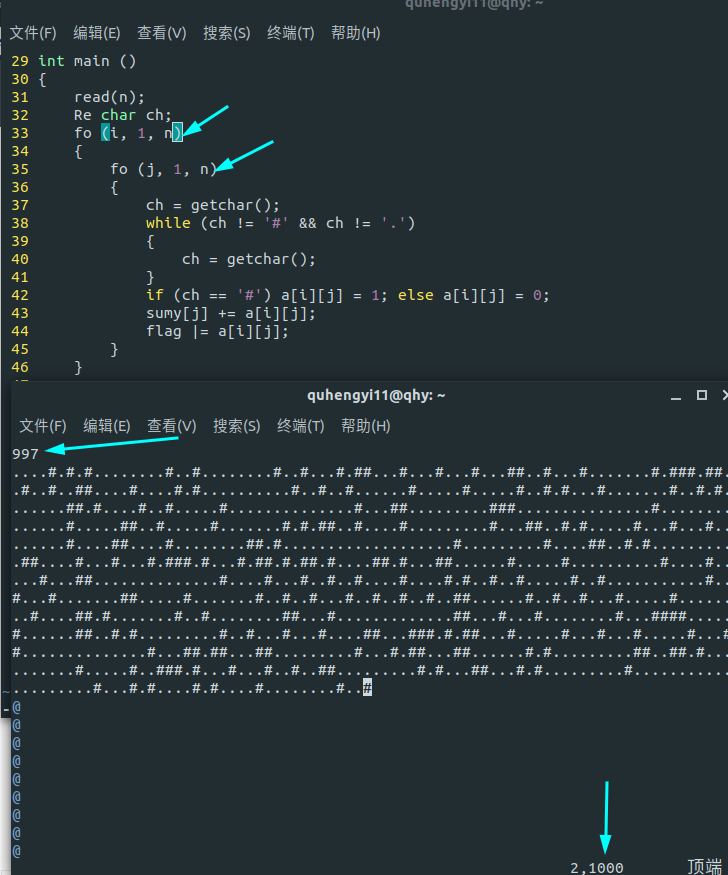### 题解#include<bits/stdc++.h>
#define Re register
#define fo(i, a, b) for (Re int i = (a); i <= (b); ++i)
#define fd(i, a, b) for (Re int i = (a); i >= (b); --i)
#define edge(i, u) for (Re int i = head[u], v = e[i].v; i; i = e[i].nxt, v = e[i].v)
#define pb push_back
#define F first
#define S second
#define ll long long
#define inf 1000000007
#define mp std::make_pair
#define mod 2000000007
#define eps 1e-4
#define lowbit(x) (x & -x)
#define N 1005
#define ls (u << 1)
#define rs (u << 1 | 1)
#define cl(arr) memset(arr, 0, sizeof arr)
{
x = 0;
Re char ch = getchar();
while (!isdigit(ch)) ch = getchar();
while (isdigit(ch)) x = (x << 3) + (x << 1) + ch - '0', ch = getchar();
}
int a[N][N], sumy[N], res, ans = inf, n;
bool flag = 0;
char s[N];
int main ()
{
Re char ch;

fo (i, 1, n)
{
scanf("%s", s + 1);
fo (j, 1, n)
{
//            ch = getchar();
//          while (ch != '#' && ch != '.') ch = getchar();
a[i][j] = (s[j] == '#');
sumy[j] += a[i][j];
flag |= a[i][j];
}
}

if (!flag) { printf("-1\n"); return 0; }
fo (i, 1, n) if (sumy[i] < n) ++res;
//    printf("%d", res);
fo (i, 1, n)
{
int now = res;
bool fl = sumy[i];
fo (j, 1, n)
if (!a[i][j]) ++now;
now += (!(bool)sumy[i]);
//        printf("%d ", now);
ans = std::min(ans, now);
}
printf("%d\n", ans);
return 0;
}